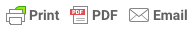# Column Layout SnippetsFollow

You can use Column Layout Snippets to create "tableless" layouts. If possible, you should use a mobile-ready content block. The snippets are useful in Event activity or category instructions/descriptions.

To utilize a snippet, you need to copy/paste the HTML below.

## 50/50 Split``````<div class="snippetrow">
<div class="split50left">
Your content goes here. This is the left column.
</div>
<div class="split50right">
Your content goes here. This is the right column.
</div>
</div>``````

## 60/40 Split``````<div class="snippetrow">
<div class="split60left">
Your content goes here. This is the left column.
</div>
<div class="split40right">
Your content goes here. This is the right column.
</div>
</div>``````

## 40/60 Split``````<div class="snippetrow">
<div class="split40left">
Your content goes here. This is the left column.
</div>
<div class="split60right">
Your content goes here. This is the right column.
</div>
</div>``````

## 70/30 Split``````<div class="snippetrow">
<div class="split70left">
Your content goes here. This is the left column.
</div>
<div class="split30right">
Your content goes here. This is the right column.
</div>
</div>``````

## 30/70 Split``````<div class="snippetrow">
<div class="split30left">
Your content goes here. This is the left column.
</div>
<div class="split70right">
Your content goes here. This is the right column.
</div>
</div>``````

## 80/20 Split``````<div class="snippetrow">
<div class="split80left">
Your content goes here. This is the left column.
</div>
<div class="split20right">
Your content goes here. This is the right column.
</div>
</div>``````

## 20/80 Split``````<div class="snippetrow">
<div class="split20left">
Your content goes here. This is the left column.
</div>
<div class="split80right">
Your content goes here. This is the right column.
</div>
</div>``````

## 3 Column Split``````<div class="snippetrow">
<div class="split33left">
Your content goes here. This is the left column.
</div>
<div class="split33middle">
Your content goes here. This is the middle column.
</div>
<div class="split33right">
Your content goes here. This is the right column.
</div>
</div>``````

## 4 Column Split``````<div class="snippetrow">
<div class="split25colA">
Your content goes here. This is the left column (A).
</div>
<div class="split25colB">
Your content goes here. This is the second from the left column (B).
</div>
<div class="split25colC">
Your content goes here. This is the third from the left column (C).
</div>
<div class="split25colD">
Your content goes here. This is the far right column (D).
</div>
</div>``````

## 5 Column Split``````<div class="snippetrow">  <div class="split20colA">
Your content goes here. This is the left column (A).
</div>
<div class="split20colB">
Your content goes here. This is the second from the left column (B).
</div>
<div class="split20colC">
Your content goes here. This is the third from the left column (C).
</div>
<div class="split20colD">
Your content goes here. This is the fourth from the left column (D).
</div>``````  <div class="split20colE">
Your content goes here. This is the far right column (E).
</div>
</div>``````

## 6 Column Split``````
<div class="snippetrow">
<div class="split16colA">
Your content goes here. This is the left column (A).
</div>
<div class="split16colB">
Your content goes here. This is the second from the left column (B).
</div>
<div class="split16colC">
Your content goes here. This is the third from the left column (C).
</div>
<div class="split16colD">
Your content goes here. This is the fourth from the left column (D).
</div>
<div class="split16colE">
Your content goes here. This is the fifth from the left column (E).
</div>
<div class="split16colF">
Your content goes here. This is the far right column (F).
</div>
</div>``````

Do you want to add some spacing above and below your content? Easy, just wrap your content in a div tag and then add one of the following classes to it. Your HTML should look something like the following.
 Top Bottom Left Right ``````paddingtop10 paddingtop20 paddingtop30 paddingtop40 paddingtop50`````` ``````paddingbottom10 paddingbottom20 paddingbottom30 paddingbottom40 paddingbottom50`````` ``````paddingleft10 paddingleft20 paddingleft30 paddingleft40 paddingleft50`````` ``````paddingright10 paddingright20 paddingright30 paddingright40 paddingright50``````